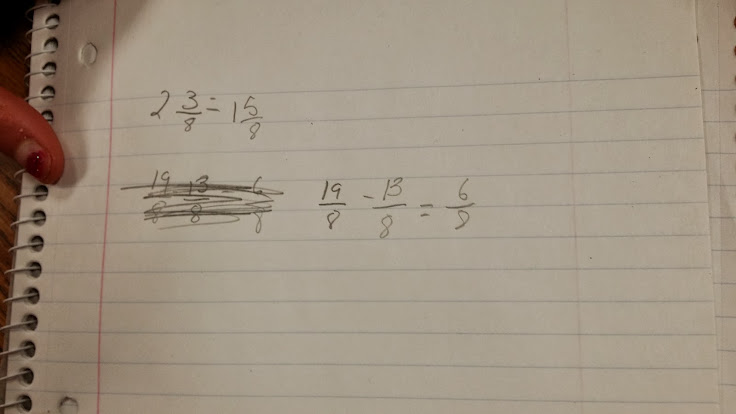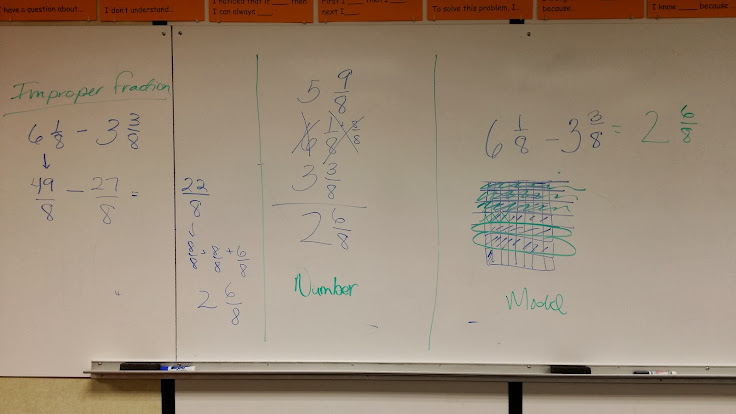# Cats and Strawberries - Inquiry with Subtracting Mixed Numbers

22 teachers like this lesson
Print Lesson

## Objective

SWBAT explore strategies for subtracting mixed numbers with like denominators.

#### Big Idea

In this lesson, students review strategies for adding mixed numbers and then work through how to subtract mixed numbers in an inquiry type of approach.

## Number Hook - Magic Trick

7 minutes

I use a number trick called Hailstone numbers to engage students before today's lesson.

I ask students to write down a number between 7 and 20.  Then they will do one of two things:

• if it is even divide it by 2,
•  if odd multiply by 3 and add 1 then keep going,

I ask students to keep track of the sequence of numbers that they generate. For example, if a student started with 7, and followed the above rules, the sequence would look like this:

7 -> 22 -> 11 -> 34 -> 17 -> 52 -> 26 -> 13 -> 40 -> 20 -> 10 -> 5 -> 16 -> 8 -> 4 -> 2 -> 1.

It seems no matter what number you start with you eventually hit a 1. These sequences are called the "hailstone numbers" because, like hailstones, they go up and down a number of times before inevitably falling to Earth.

My students noticed that if they started with the numbers 8 or 16, they only had to divide and they never got "odd" numbers in which they were required to multiply by three and add one.  I asked students if they noticed anything about the numbers.  Many students noted that they were multiples of 8, since 16 was the double of 8.  I then asked what other numbers they would predict, following the above rules, would result in only division.   Many students actually shouted excitedly 32.  When I asked them why, they said because 32 is the double of 16 and it's also a multiple of 8.  So we all tried it together. The number sequence for 32 looks like this.

32 --> 16--> 8--> 4--> 2--> 1

My students then wanted to try 64.  And YES, it works too!

## Concept development

30 minutes

To begin this lesson I quickly review the different strategies students have previously listed for adding mixed numbers.

I tell students that today they will work with a partner to subtract mixed numbers and together they will come up with their own strategies for how to subtract mixed numbers.

I then give students several subtraction problems to solve.   I allow them to use any manipulative materials they choose to help them think about the problem by circulating around the room as partners solve the problems.   I also ask students to draw visual fraction models or representations to help them think about the situations.

The problems students work on are below. You can also access them here. - subtracting mixed numbers in context.docx

1. I have 4 1/4cups of cat food.  My cat eats 2/4 of a cup.  How much cat food is left?

In this video you can hear and see two student working on this problem.  They both started with a visual fraction model  but arrived at different answers. If you look closely you can see that one student subtracted 3/4 instead of 2/4.  She didn't discover this in the video, but when another student was sharing his/her strategy at the board, she very excitedly yelled out, I figured it out.  While some teachers may be irritated with a distraction like this, I delight in them. She learned from her own mistake which is so powerful.

2. A carpenter has a board that is 6 1/3  feet long.  He cut off  2 2/3   feet.  How much is left?

3. My friend walked 1  4/5 miles.  I walked 2/5  miles less.  How far did I walk?

4. I need 2  3/8 pounds of strawberries for a pie.  I have 1  5/8 pounds.  How many more pounds of strawberries do I need?

You can see in this photo below a student who has discovered he/she can turn the mixed numbers into improper fractions and then subtract.  I have not taught my students any methods or strategies prior to this lesson about how to subtract mixed numbers. This lesson is so powerful because it gives students opportunities to make sense of the problems for themselves.  To stand at the board and deliver a list of procedures for students to do in order to subtract mixed numbers doesn't allow students to deepen their understanding of fractions or build their conceptual understanding of mixed numbers.Students spend the majority of the lesson wrestling with the problems and discovering strategies for subtracting mixed numbers.

## Student debrief - Wrap up

20 minutes

During this wrap up, I lead a strategy discussion and asked student partners to share answers and HOW they arrived at that answer. This can be a time consuming process and it is important that I keep the conversations lively, and focused. One way I try to get students to stay focused is by asking them to repeat a strategy that another student shared.

I lead a discussion and draw on the board the strategies that students have come up with.  You can see in this photo the strategies I've listed: improper fractions, number methods, and models.